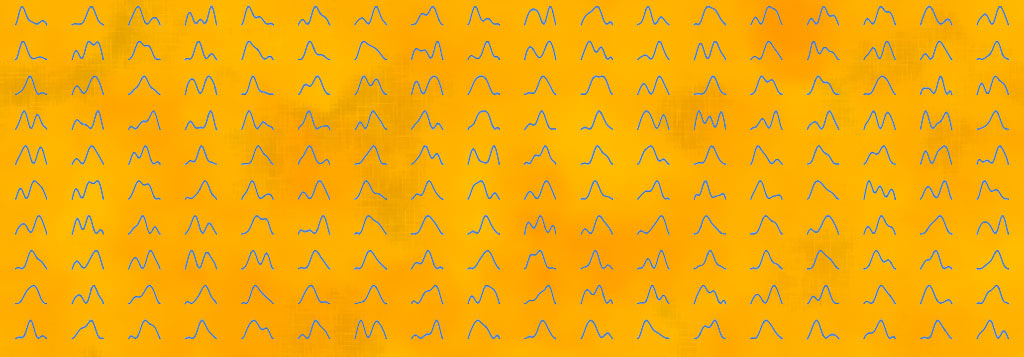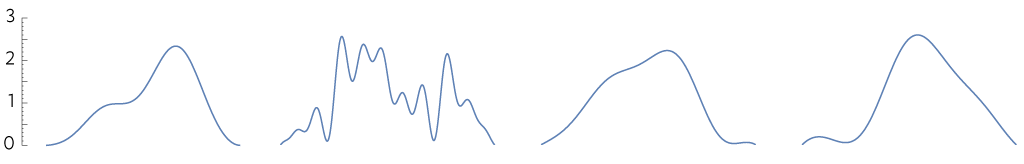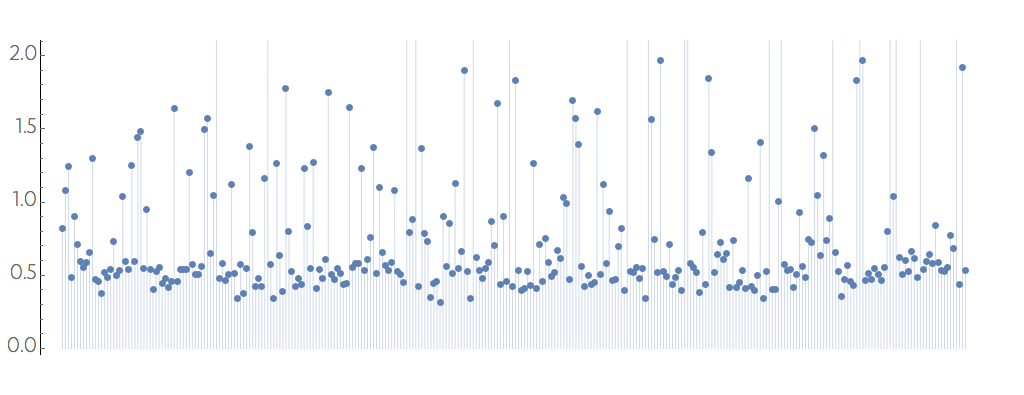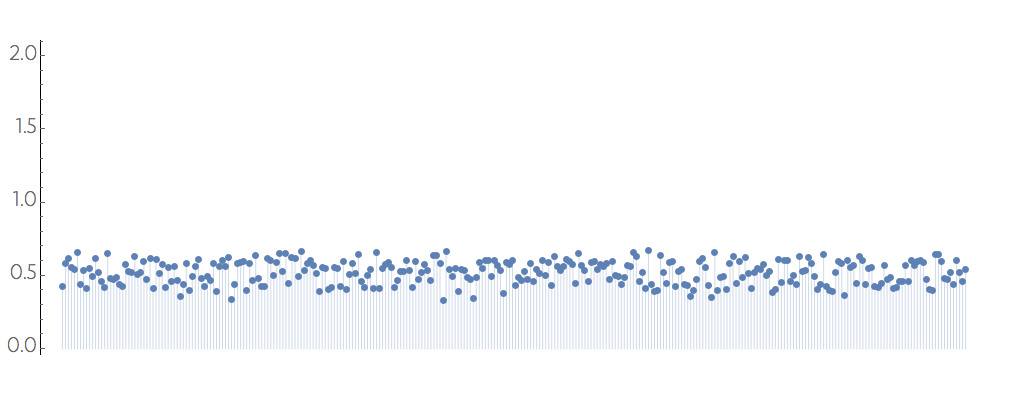# Markov's boundI find it much easier to understand math from another person than from just reading it. One of my theories of why, is that the painful and tortuous way something gets understood is not the way it gets written up. In an attempt to expose that even simple things are not always that obvious, I captured the path I had taken while noodling over the Markov inequality by going over all the paper in my wastebasket. I did not write the little applet below, but I did something like that on paper. The linked Mathematica notebook is just a commented version of what I did while thinking about the inequality.

Markov’s inequality is a fundamental lemma for a class of results known as Chernoff bounds, which play an important role in studying the curse of dimensionality and rare events. Chernoff bounds are upper bounds for how much probability is in the tail of a distribution. The ideas around the proof of these inequalities offer insights into the behavior of high-dimensional spaces and rare events.

Chernoff’s work from 1952 sparked a large literature of related results that have found applications in statistical mechanics, where large deviations relate to the free energy, to machine learning, where they help control errors, or wherever randomness and structure intermingle. Another strand of work stems from the work of Erdös and collaborators, some of it displayed in the very elegant book, The probabilistic method by Noga Alon and Joel Spencer.

The first ingredient in understanding a Chernoff bound is Markov’s inequality, which tells you that the probability of a non-negative random variable $X$ being larger than some positive multiple $t$ of its mean $\mathbb{E}[X]$ is always less than $1/t$:

$$\mathbb{P}[X > t \, \mathbb{E}[X]] < \frac{1}{t}$$

If $t<1$ the inequality is not very useful, as $\mathbb{P}[\cdot]$ is always smaller than 1. With $t=2$ my intuition of symmetric probability distributions kicks in and it seems natural that the chance of any event $X$ being, say, twice as large as the mean is never more than 12, after all, only half of the probability is to the right of the mean. But, with $t=3$, why $1/3$? And what if it is not symmetric?

## Intuition

Imagine we have a distribution with only a finite number of possible values $x_i$ for the random variable $X$. If the value $x_i$ has probability $\rho_i$, the average $\mathbb{E}[X]$ is computed by the sum

$$\mathbb{E}[X] = \sum_i \rho_i x_i$$

Notice that in the inequality, the $t$ is a scale to the mean $\mathbb{E}[X]$. It is as if we used $\mathbb{E}[X]$ as the unit of measurement for $X$. To gain some intuition, we will try to “defeat” the inequality by creating a “bad” $\rho$.

If all the mass is near the mean, then it is easy to satisfy the inequality. The hard part happens when $\rho$ has as much mass as possible away from zero. But we cannot arbitrarily place all the probability mass. However we distribute it, we must do so while keeping the average fixed. What matters for the average are the products $\rho_i x_i$ so if we multiply $x_i$ by some number the term $\rho_i$ has to be divided by that number to keep the average fixed. That makes it harder to move much mass to the tail of the distribution. The problem behaves exactly as a level seesaw. Try it:

Drag the probability bars to the right of the mean beyond the tail marker. Drag the tail marker to measure different regions. Press t to toggle the tail marker.

As the probability bars move to the tail of the distribution they get shorter. There is a small amount of rescaling that needs to happen, because not only does the mean remain constant in the seesaw, so should the total amount of probability. If after manipulating the bars, you end up with, say, $0.18$ of the distribution to the right of the mean, then $t$ could be as big as $1/0.18 = 5.56$ and the result would still hold.

## Saturation

Another way to understand an inequality is to see how close it gets to being an equality. The reverse is common strategy when dealing with complicated structures: when we cannot solve the complicated case, we may still be able to bind it by a simpler problem that we can solve. Hopefully the two are not too different. To test this out, we create some random probability distributions $\rho$, pick a $u$ uniformly between 0.1 and 0.9, and compare the actual probability

$$\mathbb{P}[X>u] = \int_{u}^{\infty} \rho(x) dx$$

with $t = u/\mathbb{E}[X]$ by subtracting one from the other, $\mathbb{E}[X]/u - \int \rho$. I made up a process that generates these probabilities over the unit interval (details in the Mathematica notebook)and from 300 of such probabilities I computed the difference between the estimate for the probability in the tail and the actual value of that probability:Smaller is better: if the estimate offered by the Markov inequality is sharp, the difference would be zero. Playing with the seesaw gives the intuition that the estimate gets better the further out the $u$ is on the tail of the distribution. Here is the same simulation, but now with $0.85 < u < 0.90$:## Proof

The proof of Markov’s inequality is done through algebraic manipulations that exploit the linearity of the expectation function $\mathbb{E}[\cdot]$ and the positivity of $X$.

We start by noting the tautology:

$$\begin{split} \mathbb{E}[x] & = \int_0^\infty dx \, x \rho(x) \\[2ex] & = \int_0^u dx \, x \rho(x) + \int_u^\infty dx \, x \rho(x) \end{split}$$

The first term of the sum is an integral of positive quantities, so if we throw it away the sum should be smaller.

$$\mathbb{E}[x] \ge \int_u^\infty dx \, x \rho(x)$$

In the remaining term, the smallest $x$ will ever be is $u$, so if we replace the quantity in the integrand by $u$ the right hand becomes even smaller. Also, $u$ is a constant, so we can move outside the integral

$$\mathbb{E}[x] \ge u \int_u^\infty dx \, \rho(x)$$

The integral is the probability of the event $X>u$, so we can re-write it as

$$\mathbb{E}[x] \ge u \, \mathbb{P}[ x > u ]$$

Now just rename $\mathbb{E}[x]/u = 1/t$ and we have the result.